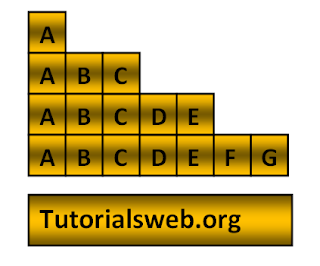## Write "C"  Program to print below Alphabet Pattern11 (or) Character Pattern11:To understand this example, you should have knowledge of the following topics:
·     C programming operators
C Character pattern11 program using For loop:
```
#include<stdio.h>
void main()
{
int i,j,k;
printf("Enter the number of lines\n");
scanf("%d",&k);
for(i = 1;i <= k;i++)
{```
```        for(j = 1;j <= (i*2-1);j++)
{```
`           printf("%c",(char)(j+64));`
```        }
printf("\n");
}
}
```

Output:
```
Enter the number of lines
5
A
ABC
ABCDE
ABCDEFG
ABCEFGHI
```
Explanation:
• First three integers "i", "j", "k"  are declared of type int.
• Then in the First for loop “i” value is initialized with a value of "1".
• Now “i” value is checked with the condition "i <= n" (where "n" value is given through Keyboard). If the condition is True loops enter into second for loop.
• Now the loop enters into second for loop and checks the condition j <=i. Where "j = 1". and the condition is True.
• Now prints the " Alphabet". Print up to second for loop condition becomes False.
• When the value of "i"  becomes greater than "k" value the program terminates.

## Search This Blog

### C Data Types and Types of data types in C

Data types specify the type of data that a variable can store. All C compilers support Five fundamental data types, they are an integer(int...